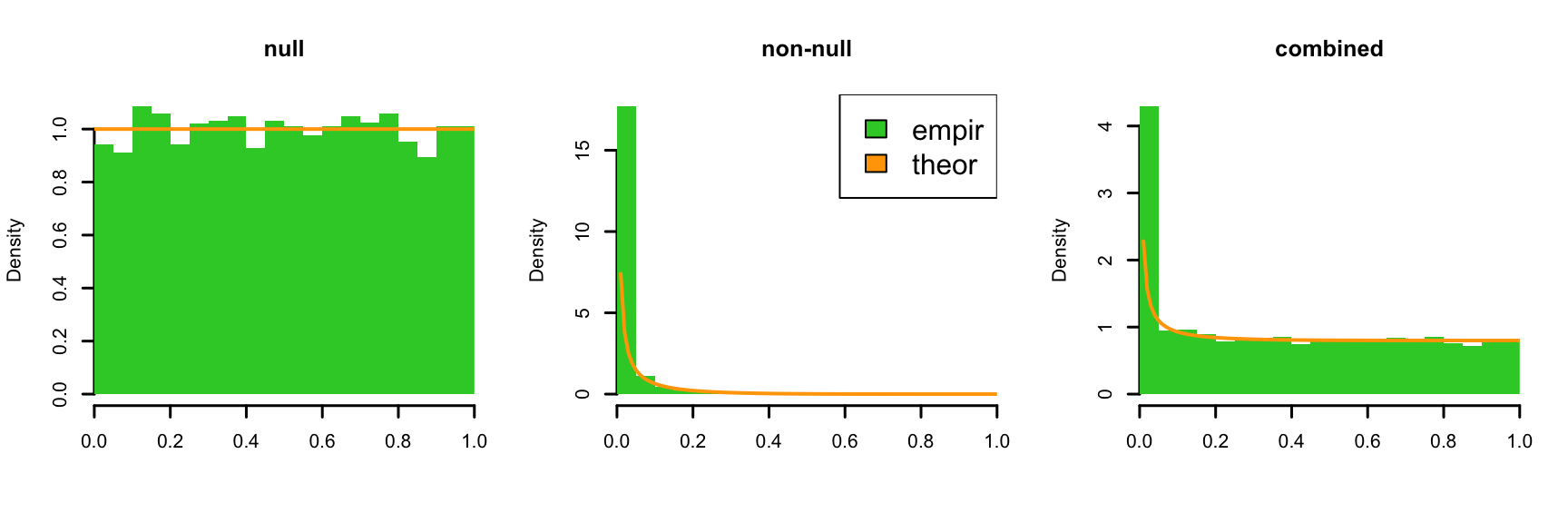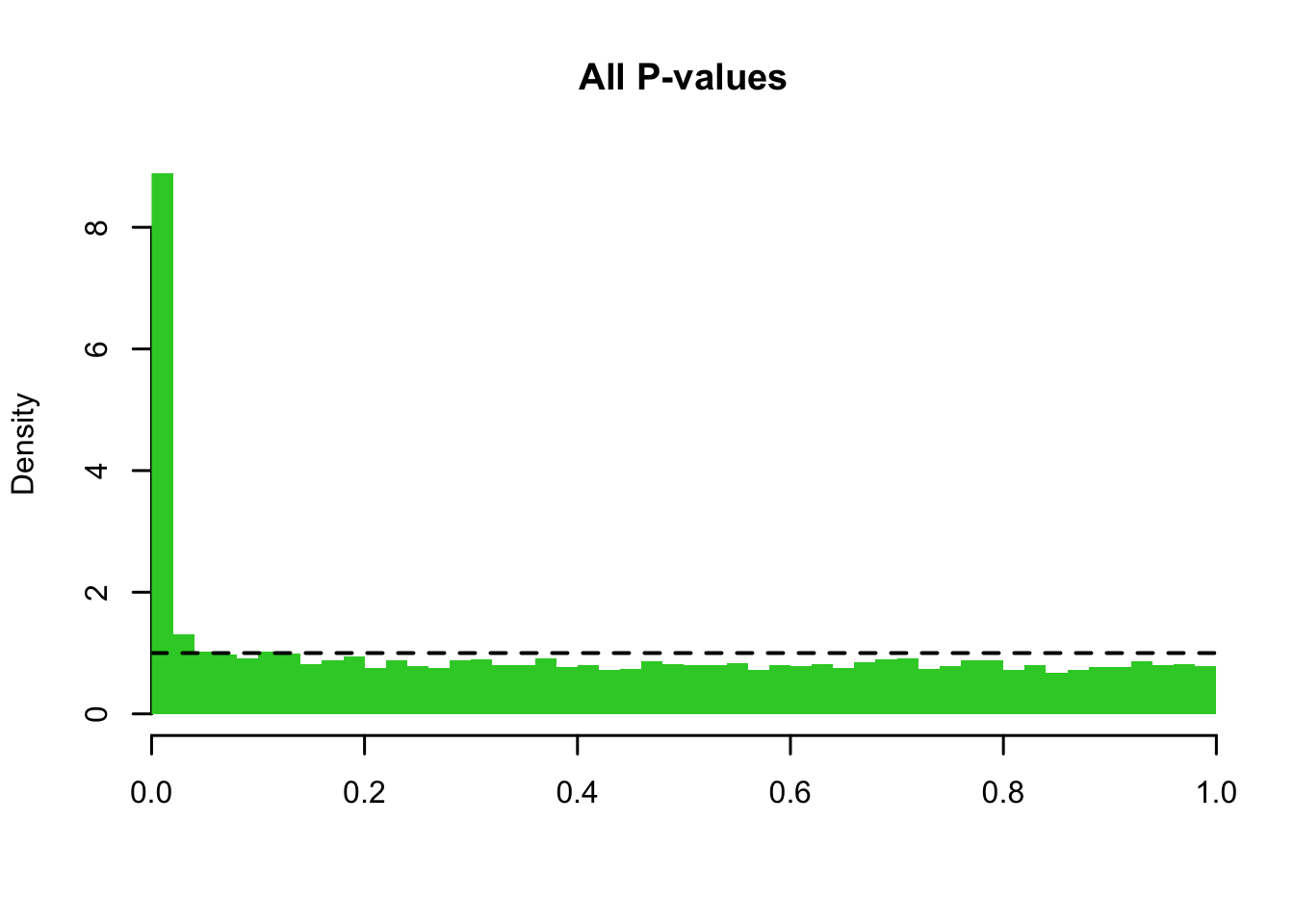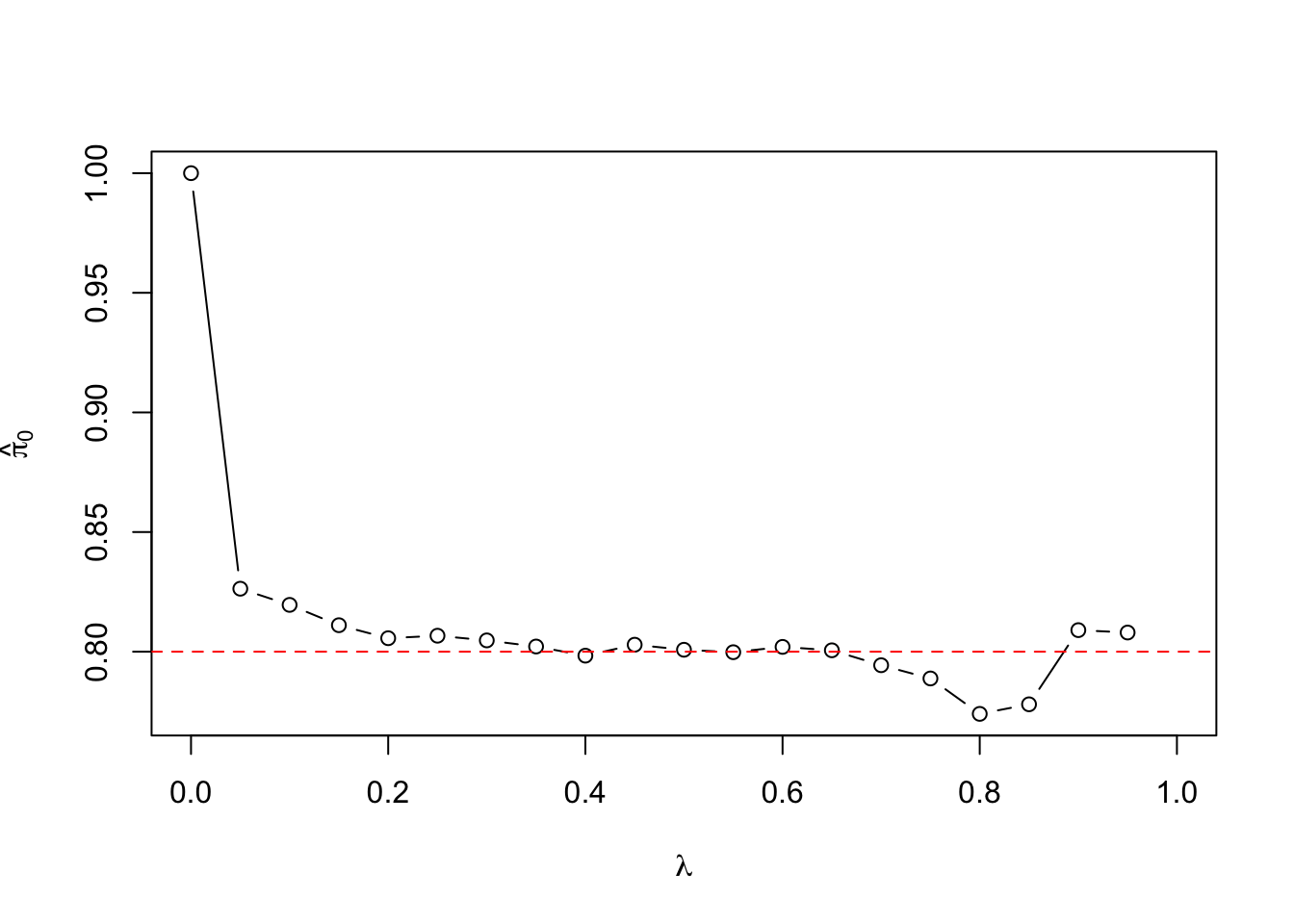So far we have used P-values for inference based on false positive rate (this is the definition of P-value itself), on family-wise error rate (FWER; Bonferroni and Holm) and on false discovery rate (FDR; Benjamini-Hochberg and Benjamini-Yekutieli). See slides.

Here we will consider a quantity called Q-value that can be attached to each test and that gives an empirical estimate of FDR among all tests whose Q-values are smaller or equal to the given Q-value. We will follow Storey and Tibshirani (2003, PNAS 100: 9440-9445), whose work led to qvalue R-package. Before defining Q-value we will first refine BH method by empirically estimating $$p_0$$, the number of null tests. Remember that the proof of BH theorem showed that BH($$\alpha_F$$) method actually controls FDR at level $$p_0/p \cdot \alpha_F.$$ When $$p_0$$ is considerably smaller than $$p$$, we can improve the accuracy of the FDR method by estimating $$p_0.$$

Let’s define, for each P-value threshold $$t\in[0,1],$$ $\textrm{FDR}(t) = \textrm{E}\left(\frac{\textrm{FD}(t)}{D(t)}\right),$ where random variables $$\textrm{FD}(t)=\#\{\textrm{null P-values} \leq t\}$$ and $$D(t)=\#\{\textrm{P-values} \leq t\}$$ in an experiment where in total $$p$$ P-values are available.

To refine our understanding of FDR methods, let’s think that random variables $$\textrm{FD}(t)$$ and $$D(t)$$ result from $$p$$ draws of P-values from a mixture distribution between Uniform(0,1) (for null P-values) and an alternative distribution with cdf $$\Phi_1$$ and pdf $$\phi_1$$ (for non-null P-values), with mixture proportion $$\pi_0$$ for the null. In other words, cdf $$\Phi$$ and pdf $$\phi$$ of the P-values are $\begin{eqnarray} \Phi(t)&=&\pi_0 \cdot t + (1-\pi_0) \Phi_1(t), \qquad t\in[0,1],\\ \phi(t)&=&\pi_0 \cdot 1 + (1-\pi_0) \phi_1(t), \qquad t\in[0,1]. \end{eqnarray}$ We can interpret sampling from such a mixture distribution as a 2-step process. Namely, we first choose between the null distribution (with probability $$\pi_0$$) and the alternative distribution (with probability $$1-\pi_0$$), and second, conditional on the chosen distribution, we sample a P-value from the chosen distribution.

Example 3.1. Suppose we do $$p=10000$$ tests of which $$m=2000$$ are non-null, i.e., we can model this as a mixture distribution with $$\pi_0=\frac{p-m}{p} = 0.80$$. Null P-values come from Uniform(0,1) and non-null P-values come from distribution Beta(0.1,4.9). (Beta(0.1,4.9) gives values in [0,1] that have mean $$0.1/(0.1+4.9)=0.02$$ and a skew to right; there is no particular reason why in real data non-null P-values had exactly this distribution, but this is used for a demonstration purpose here; see figures below.) Let’s plot theoretical (orange) and empirical (green) density functions for (1) null tests, (2) non-null tests and (3) for all tests combined (that is, the mixture distribution of the null and alternative distributions).

p = 10000
m = 2000
beta.1 = 0.1 # weight for unit interval's end point 1
beta.0 = 4.9 # weight for unit interval's end point 0
null.pval = runif(p-m, 0, 1)
alt.pval = rbeta(m, beta.1, beta.0) #non-null = alternative distribution
pval = c(alt.pval, null.pval) #all P-values together
eff = c(rep(T, m), rep(F, p - m)) #indicator for non-null effects

par(mfrow = c(1,3)) #Empirical histogram and theoretical curve for (1), (2), (3)
hist(null.pval, breaks = 20, prob = T, col = "limegreen", main = "null", xlab = "",
xlim = c(0,1), sub="", lwd = 1.5, lty = 2, xaxs="i", border = NA)
curve(dunif(x, 0, 1), 0, 1, add = T, col = "orange", lwd = 2)

hist(alt.pval, breaks = 20, prob = T, col = "limegreen", main = "non-null", xlab = "",
xlim = c(0,1), sub="", lwd = 1.5, lty = 2, xaxs="i", border = NA)
curve(dbeta(x, shape1 = beta.1, shape2 = beta.0), 0, 1, add = T, col = "orange", lwd = 2)
legend("topright", fill = c("limegreen","orange"),
legend = c("empir","theor"), cex = 1.5)

hist(pval, breaks = 20, prob = T, col = "limegreen", main = "combined", xlab = "",
xlim = c(0,1), sub="", lwd = 1.5, lty = 2, xaxs="i", border = NA)
curve((p-m)/p*1 + m/p*dbeta(x, shape1 = beta.1, shape2 = beta.0), 0, 1, add = T,
col = "orange", lwd = 2)Density plots show how the null P-values follow the uniform distribution and non-null P-values are enriched for small values near 0.

summary(null.pval)
##      Min.   1st Qu.    Median      Mean   3rd Qu.      Max.
## 0.0000108 0.2521960 0.4999947 0.5002426 0.7467176 0.9998005
summary(alt.pval)
##      Min.   1st Qu.    Median      Mean   3rd Qu.      Max.
## 0.0000000 0.0000001 0.0001608 0.0196240 0.0081581 0.7202532

When these two distributions are combined in the rightmost panel, the enrichment of small P-values is still present but has a smaller weight of 20% compared to the weight of 80% given to the null distribution.

Since $$\Phi(t)$$ is the probability that a particular P-value from this mixture distribution $$\leq t$$, the random variables $$D(t)$$ and $$\textrm{FD}(t)$$ are distributed as $\begin{eqnarray} D(t) &\sim& \textrm{Bin}(p,\Phi(t)) \\ \textrm{FD}(t)\,|\,D(t)&\sim& \textrm{Bin}(D(t), \theta_t), \text{ where } \\ \theta_{t} &=& \textrm{Pr}(\textrm{NULL} \, | \, P \leq t ) = \frac{\textrm{Pr}(\textrm{NULL})\textrm{Pr}(P \leq t \, | \, \textrm{NULL})}{\textrm{Pr}(P \leq t)}\\ &=&\frac{\pi_0 t} {\pi_0 t + (1-\pi_0)\Phi_1(t)}. \end{eqnarray}$ Using the law of total expectation: $$\textrm{E}_Y(Y) = \textrm{E}_X(\textrm{E}_Y(Y\,|\,X))$$, we have that $\textrm{FDR}(t) = \textrm{E}\left(\frac{\textrm{FD}(t)}{D(t)}\right)= \textrm{E}\left(\textrm{E}\left(\frac{\textrm{FD}(t)}{D(t)}\,\middle|\,D(t)\right)\right)= \textrm{E}\left(\frac{1}{D(t)} \textrm{E}(\textrm{FD}(t)\,|\,D(t)) \right)= \textrm{E}\left(\frac{1}{D(t)} \theta_{t}\,D(t) \right)= \theta_{t}.$ On the other hand, $$\textrm{E}(\textrm{FD}(t)) = \textrm{E}(\textrm{E}(\textrm{FD}(t)\,|\,D(t))) =\textrm{E}(D(t)\theta_t) = \theta_t\cdot \textrm{E}(D(t))$$ Thus $\frac{\textrm{E}(\textrm{FD}(t))}{\textrm{E}(D(t))} = \frac{\theta_t\cdot\textrm{E}(D(t))}{\textrm{E}(D(t))} = \theta_t= \textrm{FDR}(t).$ So we can estimate $$\textrm{FDR}(t)$$ by the ratio of expectations of $$\textrm{FD}(t)$$ and $$D(t)$$. And what could we use as estimates for these expectations?

For each P-value threshold $$t$$, denote the number of all discoveries at threshold $$t$$ by $$\widehat{D}(t) = \#\{\textrm{P-values} \leq t\}.$$ We use this to estimate $$\textrm{E}(D(t)) \approx \widehat{D}(t)$$.

To estimate $$\textrm{E}(FD(t))$$, we remember that the null P-values are uniformly distributed and hence $$\textrm{E}(FD(t)) = p_0 \cdot t = \pi_0\cdot p \cdot t.$$ In estimating $$\pi_0$$ we again rely on the fact that the null P-values are uniformly distributed and that most P-values near 1 are expected to be from the null distribution. Let’s remind us how our P-value distribution looked like.

hist(pval, breaks = 40, prob = T, col = "limegreen", main = "All P-values", xlab = "",
xlim = c(0,1), sub = "", lwd = 1.5, lty = 2, xaxs = "i", border = NA)
abline(h = 1, lty = 2, lwd = 2)We can see that the density of P-values > 0.2 looks fairly flat. If we assume that the density of non-null P-values is $$\approx 0$$ in this region, then the density function of P-values in this region is approximately $$\pi_0\cdot 1 + (1-\pi_0)\cdot 0 = \pi_0$$. Thus the value of the density function in this flat part gives an estimate of the overall proportion of null P-values $$\pi_0$$. The constant density function over any interval $$(\lambda, 1]$$ is the probability mass in the interval divided by the length of the interval ($$1-\lambda$$). With empirical data, this probability mass is
$$\#\{P_j >\lambda \,|\, j=1,\ldots,p\}/p$$. We can apply such an estimate for any value $$\lambda$$ to yield an estimator $\widehat{\pi}_0(\lambda) = \frac{\#\{P_j >\lambda\, |\, j=1,\ldots,p\}}{(1-\lambda) p}.$ Parameter $$\lambda$$ should be large enough that there are not many true effects with P-values $$>\lambda$$ but simultaneously $$\lambda$$ should be small enough that there are enough null P-values $$> \lambda$$ so that the estimate has a good precision. An inclusion of a few non-null P-values $$>\lambda$$ makes this estimate conservative, that is, an overestimate of $$\pi_0$$. That keeps us on the safe side and leads to an overestimate $$\textrm{FDR}(t)$$.

If we take $$\lambda = 0$$, then $$\widehat{\pi}_0(\lambda)=1$$ which is usually much too conservative when a considerable proportion of tests are expected to be truly non-null. On the other hand, as we set $$\lambda$$ closer to 1, the variance of $$\widehat{\pi}_0(\lambda)$$ increases due to decreasing number of P-values exceeding $$\lambda$$, which makes the estimate more unreliable. Therefore, as a simple, practical choice for $$\lambda$$ we could use 0.5, but optimal threshold would depend on the data set. Let’s see see how the choice of $$\lambda$$ affects the estimate $$\widehat{\pi}_0(\lambda)$$ in our ongoing example data.

lambda = seq(0, 1, by = 0.05)
pi.0 = sapply(lambda, function(x) {sum(pval > x)}) / p / (1 - lambda)
plot(lambda, pi.0, t = "b", xlab = expression(lambda), ylab = expression(hat(pi)))
abline(h = 1 - m/p, col = "red", lty = 2) #this is the true valueIndeed, we see that with values near $$\lambda = 0.5$$, the estimate $$\widehat{\pi}_0(\lambda)$$ is a good one whereas its quality deteriorates towards either of the endpoints of the interval.

With this estimator for $$\widehat{\pi}_0$$, we have the estimate $\widehat{\textrm{FDR}}(t) = \frac{\widehat{\textrm{FD}}(t)}{\widehat{D}(t)}= \frac{p\cdot \widehat{\textrm{Pr}}(\textrm{NULL P-value})\cdot \textrm{Pr}(P \leq t \,|\, \textrm{NULL P-value})}{\widehat{D}(t)} = \frac{p\cdot \widehat{\pi}_0 \cdot t}{\widehat{D}(t)}.$

Let’s plot our estimate $$\widehat{\textrm{FDR}}(t)$$ in orange as function of $$t$$ by using the value $$\widehat{\pi}_0(0.5)$$ estimated above. Let’s also plot a curve that corresponds to conservative assumption $$\pi_0=1$$ in red and let’s add the true empirical $$\textrm{FDP}(t)$$ curve in blue, which we are able to do since we know which P-values were simulated from the null.

pval.sorted = sort(pval)
pi.0 = pi.0[which(lambda == 0.5)]
par(mar = c(5,6,2,1)) # widen left margin for text
plot(pval.sorted, p * pi.0 * pval.sorted / (1:p), xlab = "t",
ylab = expression(paste(hat(FDR),"(t) or FDP(t)")), t = "l", col = "orange",
xlim = c(0,1), ylim = c(0,1), lwd = 1.5)
#Add line for conservative assumption pi.0 = 1:
lines(pval.sorted, p * 1 * pval.sorted / (1:p), xlab = "t",
col = "red", lwd = 1.5)